×

Search anything:

# Bit Array [Explained with example]

#### Algorithms Data Structures Interview Problems on ArrayGet this book -> Problems on Array: For Interviews and Competitive Programming

In this article, we have explained Bit Array which is a Data Structure used in various problems to represent combinatorial information in an array in a compressed way. We have presented a code example of Bit Array in Java as well.

1. Basics of Bit Array
2. Population or Hamming weight
3. Why bit array is used?
4. Bit Array in Java
5. Sparse vs Dense Bit arrays

# Basics of Bit Array

Bit Array is a data structures that compactly stores Boolean values or bits in the form of an array. The bits can be 0 or 1 only. Each bit in the bit array is independent.

For Example, 00111001 is an 8 bit array as there are 8 bits in them.

As each bit can have 2 values, so it can represent 2^8 values i.e. 256 which is the capacity of this bit array. We can have n-bit array where n can be any number.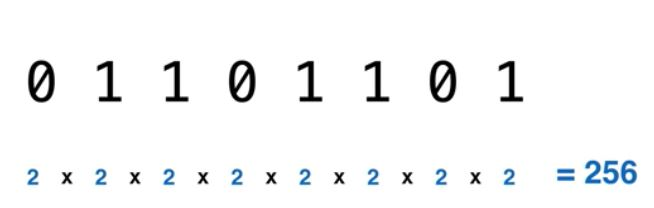A bit array is also known as bitmap, bitset and bit vectors. In java, Bit array is represented by Bitset class.

We can perform many operations on bit array like AND, OR, NOT, XOR etc.
In the picture below, Basic operations i.e. AND and OR are performed on bit array.

OR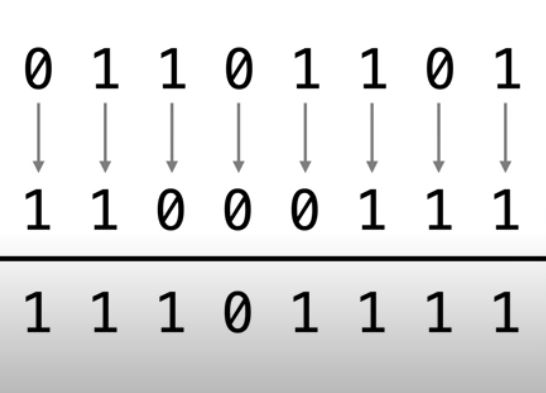AND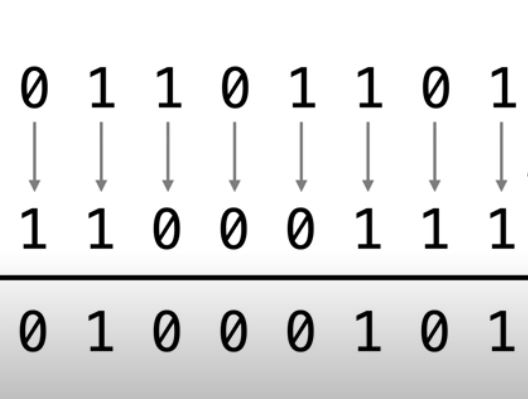# Population or Hamming weight

Population or Hamming weight of a bit array is the number of Boolean symbols that are 1. In other words, number of 1’s in the bit array is the population count of the bit array. Is is used in compression of bit array.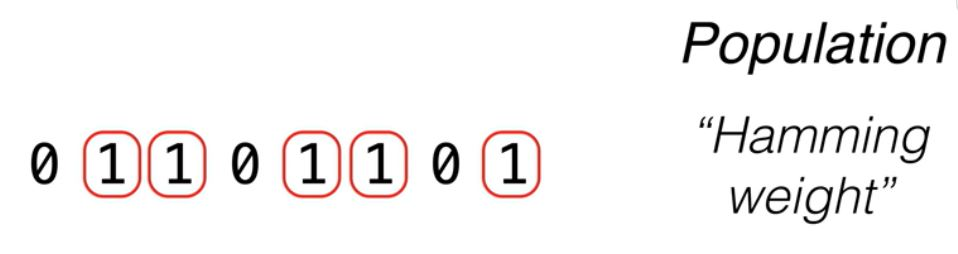# Why bit array is used?

Bit array is used to achieve bit-level parallelism in processing executions. It is a kind of parallel computing based on increasing word size of the processor which reduces the number of instructions for the processor. It allows the execution of operations to occur quickly. As a result of bit level parallelism, Bit array allows small array of bits to be stored and manipulated in the register set for long period of time.

# Bit Array in Java

BitSet class in java can be used to work with bit array. Below is a simple program in Java to use bit array and to perform basic operation(AND) on it.

``````import java.util.BitSet;

public class BitArray
{
private BitSet bit;

public BitArray(String bits)
{
this.bit = fromString(bits);
}

private void setBitSet(BitSet bitSet )
{
bit = bitSet;
}

private BitSet getBitSet()
{
return bit;
}

public BitArray and(BitArray bitarray)
{
BitSet b1 = this.getBitSet();
BitSet b2 = bitarray.getBitSet();

bits1.and(b2);
this.setBitSet(b1);
return this;
}

private BitSet fromString(String bit)
{
return BitSet.valueOf(new long[] { Long.parseLong(bit, 2) });
}

public String toString()
{
return Long.toString(bit.toLongArray(), 2);
}

public static void main (String[] arg)
{
BitArray array1 = new BitArray("1010");
BitArray array2 = new BitArray("1001");

System.out.println("The BitArray Are");
System.out.println("First :" + array1);
System.out.println("Second :" +array2);

System.out.println("After performing AND operation on First and Second");
System.out.println(array1.and(array2));
}
}
``````

Output of the above program would be :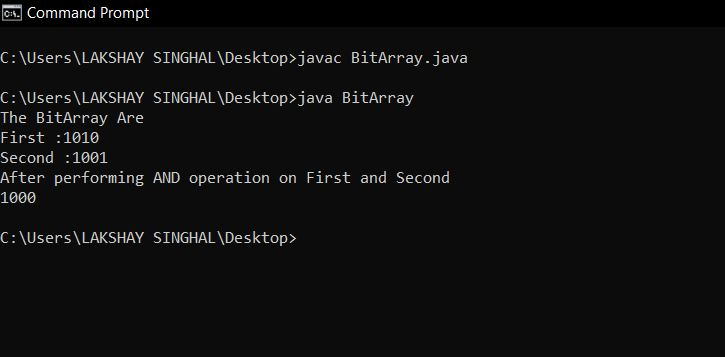# Sparse Vs Dense Bit arrays

Bit array is dense when each bit is equally likely to be 0 or 1 i.e 50% chance on being 1 or 0. They can’t be compressed much but mostly the data is not random and can be compressed. When the data is not equally likely to be 0 or 1, it is called sparse arrays and they can be compressed.

Run length encoding can be used to compress these sparse arrays.

## Question

#### What is the representation of an 8 bit array 11111111 in Decimal?

255
256
0
1
255 = 2^8 – 1

With this article at OpenGenus, you must have a good idea of Bit Array with the code example in Java.